# 新词发现

• “发现”：依据某种手段或方法，从文本中挖掘词语，组成新词表；
• “新词”：借助挖掘得到的新词表，和之前已有的旧词表进行比对，不在旧词表中的词语即可认为是新词。

“新词发现”的难点主要在于“发现”的过程——如何从文本中挖掘到词语？那么有办法回避这个问题吗？让我们思索一下“新词”的过程：比对挖掘得到的新词表和旧词表，从代码的角度来说。

for 新词 in 新词表:
if 新词 not in 旧词表:
print("这是新词")


## 基于分词的方法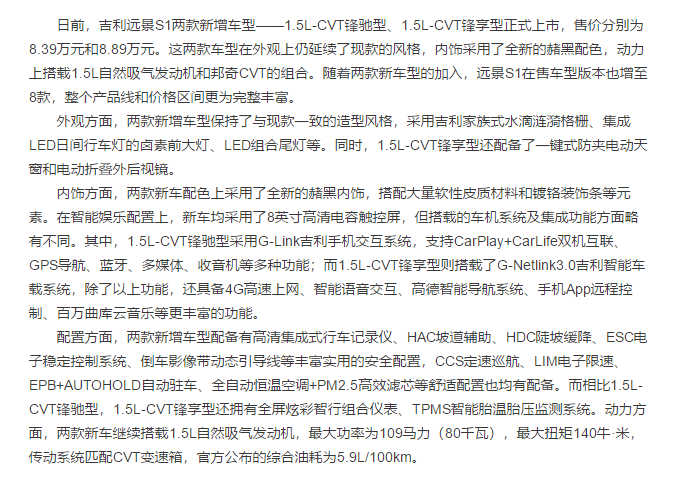【问题 1】：怎么去判断片段是否成功匹配了呢？

示例 1：内饰方面，两款新车配色上采用了全新的赭黑内饰，



【问题 2】：对于一些较长的新词，例如“人艰不拆”，使用 jieba 分词得到 [“人艰”, “不”, “拆”]，此时的未成功匹配片段就不全是单字。换言之，新词中包含词语。

【问题 3】：如果新词中包含的词语在常用词表中该怎么办？该问题相当于在问题 3 的基础上提出了——新词中包含常用词语。例如“一带一路”，使用 jieba 分词可得到 [“一带”, “一路”]。而对于问题 3 则比较难处理，需要更多繁复的规则去做后处理。

## 基于规则的方法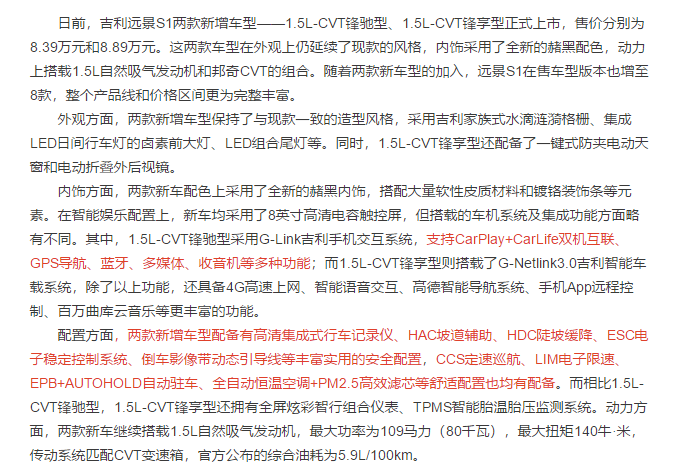【优点】：新词挖掘的效果非常好，像“支持CarPlay+CarLife双机互联”这一类夹杂中文、英文和标点符号的词语（短语）也能抽取出来。

【缺点】：与领域耦合过深，无法将建立的规则库、专业词库以及模式库迁移到其他领域中，需要根据领域内容重新搭建。甚至与特定类型、出版社、渠道的文章耦合过深，例如汽车之家的汽车文章和易车的汽车文章的格式就不同，需要为不同的数据源搭建相应的规则库。一旦数据源的文章格式变动，规则库也需要作相应的修改。无论是搭建还是后续的维护，都需要大量的人工成本。

# 基于统计的方法

## 有监督方法

• 基于文本片段的某些统计量，以此作为特征训练二分类模型；
• 基于序列信息进行序列标注直接得到新词，或对得到的新词再进行判定。

1. 构建词库
2. 新词比对

## 构建词库

• 词频
• 凝聚程度
• 自由程度

### 凝聚程度

P M I ( x , y ) = log ⁡ 2 p ( x , y ) p ( x ) p ( y ) P M I(x, y)=\log _{2} \frac{p(x, y)}{p(x) p(y)}

p(x, y) <= p(x)、p(x, y) <= p(y)，且 p(x) < 0、p(y) < 0，因此：
p ( x , y ) p ( x ) p ( y ) ≥ 1 \frac{p(x, y)}{p(x)p(y)} \geq 1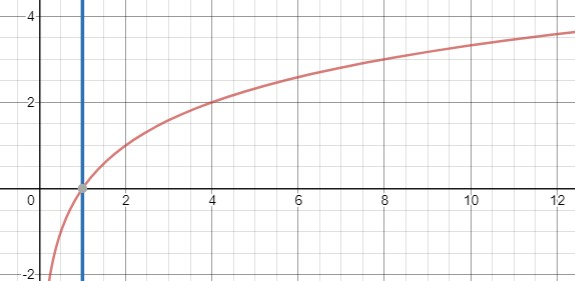• 的电影 = 的 + 电影
• 电影院 = 电影 + 院
电影：0.01，院：0.01，电影院：0.001
P(电影院)/P(电影)P(院)：0.001 / (0.01 * 0.01) = 10

P(的电影)/P(的)P(电影)：0.002 / (0.01 * 0.2) = 1


### 自由程度

matrix67 提出可以使用信息熵来进行度量，令当前文本片段为 x，其上文的词语为 y l y_l ，下文的词语为 y r y_r
H ( y ∣ x ) = − ∑ y p ( y ∣ x ) l o g p ( y ∣ x ) H(y|x) = -\sum_y p(y|x)log p(y|x)

L ( W ) = log ⁡ L E ⋅ e R E + R E ⋅ e L E ∣ L E − R E ∣ L(W)=\log \frac{L E \cdot e^{R E}+R E \cdot e^{L E}}{|L E-R E|}

# 文本切分

### 合词

【处理过程】：以“巧克力”为例。

1. 首先，计算“巧克”的凝聚程度，若超过阈值，则将其合并；
2. 然后计算“巧克”与“力”的凝聚程度，若超过阈值，则将其合并，得到“巧克力”。

【问题 1】：当两段相邻文本片段整合为一块新的文本片段时，我们该如何去获取这块新文本片段的频数呢？同样，三段、四段乃至 n 段文本片段整合后，该如何计算这些文本片段的频数呢？

【解决方案】：以“巧克力”为例，我们需要统计 2-gram 的频数，对于较长的词语，则需要统计 n-gram 的频数。

【问题 2】：令合词操作得到的文本片段为 A，下一段相邻文本片段为 B。随着合词操作的进行，P(A) 和 P(A, B) 一般会越来越小且越来越接近。此时，P(B) 对 PMI(A, B) 起主要影响作用。那么只要文本片段 B 是一些低频字，PMI(A, B) 的值仍然可以超过设定的阈值，从而将文本片段 A 和 B 整合得到 C，但 C 很有可能不是我们想要的新词，例如“前麦弗逊式独立悬架驱”，由于“驱”字在语料中出现的频率很低，因此极容易将“前麦弗逊式独立悬架”和“驱”进行整合，得到不期待的结果。

【解决方案】：使用平均互信息(AMI)作为凝聚程度的计算公式（参考自"新词发现"算法探讨与优化-SmoothNLP）。
A M I ( X , y ) = 1 n l o g 2 p ( X , y ) p ( x 1 ) ⋯ p ( x n ) p ( y ) AMI(X, y) = \frac{1}{n} log_2 \frac{p(X, y)}{p(x_1) \cdots p(x_n)p(y)}

A M I ( 巧克力 , 的 ) = 1 4 l o g 2 p ( 巧克力的 ) p ( 巧 ) p ( 克 ) p ( 力 ) p ( 的 ) AMI(\text{巧克力}, \text{的}) = \frac{1}{4} log_2 \frac{p(\text{巧克力的})}{p(\text{巧})p(\text{克})p(\text{力})p(\text{的})}

### 切词

【处理过程】：以“巧克力真好吃”为例。

1. 首先，计算“巧克”的凝聚程度，若超过阈值，则继续计算“克力”的凝聚程度，若仍然超过阈值，则继续计算下一对文本片段“力真”的凝聚程度；
2. 若“力真”的凝聚程度小于阈值，则进行切分“力|真”，继续计算下一对文本片段；
3. 最终可得“巧克力|真|好吃”。

## 合词、切词与凝聚程度

【合词与切词的比较】：

• 合词需要更多的统计信息，而切词仅需要 2-gram 的统计信息（计算相邻两个文本片段的凝聚程度）即可，因此切词的执行开销要远小于合词；
• 合词的过程中会遇到合词中提到的问题2，而切词不会出现该问题；

### 切词的缺陷“电”、“影”、“院”三位好朋友手拉手，“电”小朋友是一位交际花，她有许多的朋友，而人的精力总是有限的，因此“电”小朋友和“影”小朋友的玩耍时间远没有“影”小朋友和“院”小朋友来得多。但我们没有因为“电”的朋友多且和“影”一起玩耍的时间少，而认为他们俩不是朋友。因此，仅考虑相邻文本片段的切词过程计算得到的是“封闭程度”，而非凝聚程度。例如，“饕餮”、“沆瀣”等词，他们只拥有彼此。

min ⁡ { P ( a b c ) P ( a b ) P ( c ) , P ( a b c ) P ( a ) P ( b c ) } \min \left\{\frac{P(a b c)}{P(a b) P(c)}, \frac{P(a b c)}{P(a) P(b c)}\right\}

【示例】：以“利率”为例，在所有语料中“利率”出现的次数不会太多，同时“率”的出现次数略高于“利率”，那么在凝聚程度的计算公式中，“利”的出现次数对最终凝聚程度起到关键作用。但像“利”这一类高频字，会使得计算得到的凝聚程度较小，低于设定的阈值，从而使得“利率”这一类的词被切分。

## 切分问题的一些方案

• 调整凝聚程度计算公式；
• 依据自由程度进行扩展。

### 调整凝聚程度计算公式

【简单的实现方式】：
P M I ( A , B ) = l o g 2 α P ( A B ) β 1 P ( A ) ∗ β 2 P ( B ) PMI(A, B) = log_2\frac{\alpha P(AB)}{\beta_1 P(A) * \beta_2 P(B)}
α \alpha 用以提高 A、B 共现频率的权重，而 β 1 \beta_1 β 2 \beta_2 则可视为对高频字的惩罚系数。

• 非线性地增大共现频率的权重；
• 非线性地降低高频字的权重。

#### 非线性地增大共现频率的权重

P M I ( A , B ) ′ = P ( A , B ) l o g 2 P ( A , B ) P ( A ) P ( B ) PMI(A, B)' = P(A, B) log_2 \frac{P(A, B)}{P(A) P(B)}

A B = α P ( A B ) AB = \alpha P(AB)

f ( A B ) = l o g 2 α P ( A , B ) P ( A ) P ( B ) f(AB) = log_2 \frac{\alpha P(A, B)}{P(A) P(B)}

P M I ( A , B ) ′ = A B f ( A B ) PMI(A, B)' = AB f(AB)

f(AB) 可理解为共现频率的非线性权重系数，上述式子对实际的计算没有作用，提供了另一种角度的解释。

【互信息计算公式效果】：【原式效果】：1. 乘上共现频率后的凝聚程度阈值需要相应地调低，但共现频率的波动较大，如何设置凝聚程度阈值是一个问题；
2. 马太效应：较大的共现频率可以得到更高的凝聚程度，而较低的共现频率则得到更低的凝聚程度，这显然不是我们想看到的。

#### 非线性地降低高频字的权重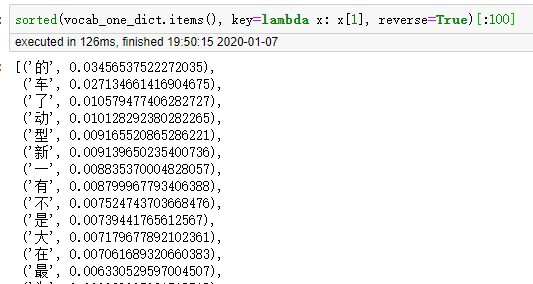'嗬', '嗯', '嗳', '嘎', '嘘', '嘛', '嘻', '嘿', ......


### 依据自由程度进行扩展

【具体步骤】：

1. 若切分后的文本片段自由程度小于设定阈值，则尝试将当前文本片段与相邻文本片段进行组合；
2. 计算组合后的文本片段的自由程度，若自由程度提高则继续组合相邻的文本片段；
3. 若连续组合之后的文本片段的自由程度高于设定阈值，则将这段文本片段作为新的词语，否则出局。

【问题 1】：连续组合相邻文本片段会导致最终得到一个超长的词语吗？

【回答 1】：不会，当超过某个临界点时（n = 4），字数越长的文本片段其自由程度越低。因为 n-gram 中的 n 越大，得到的文本的频数越低，因此其相邻词语的数量也越少，信息熵越来越低，低于设定的阈值。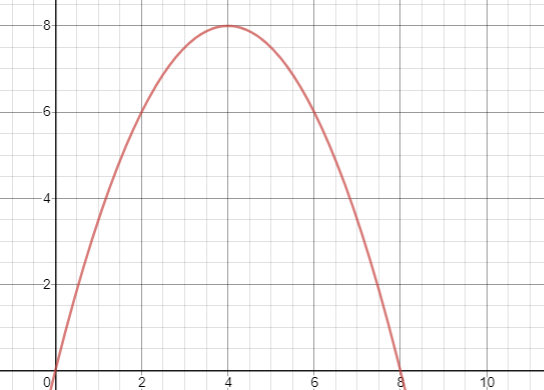【注意】：“利”的左信息熵不能作为“利率”的左信息熵，换言之，“利”的左邻词语不能作为“利率”的左邻词语，必须要“利”和“率”共有的左邻才能作为“利率”的左邻词语。右信息熵同理。

## 执行过程

1. 首先将文本拆分为文本片段（字符、英文单词等）；
2. 基于凝聚程度和切词方式对文本进行切分，获得一个粗的词库，同时也对文本做了一次粗的分词；
3. 基于自由程度从上一步的到得词库中筛选出词语；

## 告一段落

• 尝试从文本中挖掘更多的统计量，而非仅仅局限于频数和频率；
• 如何去设计一套无监督的评估指标也是非常有挑战的工作；
• 全文主要讲述切词的工作，关于合词也有不少可探索的工作。

## 参考

• 新词发现：中文新词识别技术简介：https://blog.csdn.net/sinat_33741547/article/details/81704798
• 互联网时代的社会语言学：基于 SNS 的文本数据挖掘：http://www.matrix67.com/blog/archives/5044
• "新词发现"算法探讨与优化-SmoothNLP：https://zhuanlan.zhihu.com/p/80385615
• 无监督构建词库：更快更好的新词发现算法：https://zhuanlan.zhihu=
01-1401-02
08-18332
08-158801
07-10877
03-192万+
08-06
01-054976
04-191465
11-022709
06-302688
05-29480
02-263万+
08-162万+
©️2020 CSDN 皮肤主题: 成长之路 设计师:Amelia_0503